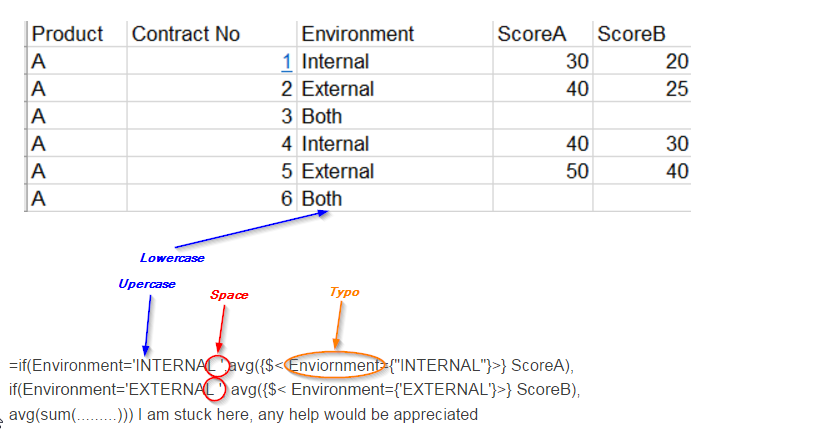Announcements
You can succeed best and quickest by helping others to succeed. Join the conversation.
cancel
Showing results for
Did you mean:Anonymous
Not applicable

## How to sum 2 fields in an IF statementIf Environ is Internal, I want the avg of Score A, If env is External, I want Avg of ScoreB, If its both, I want the avg of

scoreA and scoreB. how to write the IF statement as an expn in Straight Table.

=if(Environment='INTERNAL ',avg({\$< Enviornment={"INTERNAL"}>} ScoreA),

if(Environment='EXTERNAL ', avg({\$< Environment={'EXTERNAL'}>} ScoreB),

avg(sum(.........))) I am stuck here, any help would be appreciated

1 Solution

Accepted SolutionsMVP

=if(Environment='INTERNAL ',avg({\$< Enviornment={"INTERNAL"}>} ScoreA),

if(Environment='EXTERNAL ', avg({\$< Environment={'EXTERNAL'}>} ScoreB),

RangeAvg( avg({\$< Enviornment={"INTERNAL"}>} ScoreA),avg({\$< Environment={'EXTERNAL'}>} ScoreB))

))

9 RepliesMVP

As per above example, what is the value of ScoreA average.. ScoreB average and what is ScoreAandB both average..MVP

Finding it difficult to understand what is your expected output from the given sample?MVP

Maybe using Rangesum()?

avg( Rangesum(ScoreA, ScoreB))Anonymous
Not applicable
Author

if it is Internal env, it shoud give me an avg for (30+40) which is 35. If it is external, it should avg (25+40) which is 32.5;

if it is both it should avg the averages just caculated, which means it sud avg (35+32.5) which is 33.75MVP

May be this:

=If(Environment='INTERNAL ', Avg(TOTAL <Product> {\$< Enviornment={"INTERNAL"}>} ScoreA),

If(Environment='EXTERNAL ', Avg(TOTAL <Product>{\$< Environment={'EXTERNAL'}>} ScoreB),

RangeAvg(Avg(TOTAL <Product> {\$< Enviornment={"INTERNAL"}>} ScoreA), Avg(TOTAL <Product>{\$< Environment={'EXTERNAL'}>} ScoreB))MVP

=if(Environment='INTERNAL ',avg({\$< Enviornment={"INTERNAL"}>} ScoreA),

if(Environment='EXTERNAL ', avg({\$< Environment={'EXTERNAL'}>} ScoreB),

RangeAvg( avg({\$< Enviornment={"INTERNAL"}>} ScoreA),avg({\$< Environment={'EXTERNAL'}>} ScoreB))

))Anonymous
Not applicable
Author

Swuehl,

Thank you so much. It looks like your soln is correct. Let me plug in the changes in my qvw and I will get back to close this thread.

regards,

DCreator

swuehl, solution will work.

just another way to implement, which i read in one of the blogs works better

=pick(match(Environment,'INTERNAL','EXTERNAL','BOTH')

avg({\$< Enviornment={"INTERNAL"}>} ScoreA),

avg({\$< Environment={'EXTERNAL'}>} ScoreB),

RangeAvg( avg({\$< Enviornment={"INTERNAL"}>} ScoreA),avg({\$< Environment={'EXTERNAL'}>} ScoreB))

)Partner - Specialist

Hi QlikDash,

Sunny and Swuehl give you the correct answer. They copied and completed your expression, but your expression was not correctTheir response with corrections:

=If(Environment='Internal', Avg(TOTAL <Product> {\$< Environment={'Internal'}>} ScoreA),

If(Environment='External', Avg(TOTAL <Product>{\$< Environment={'External'}>} ScoreB),

RangeAvg(Avg(TOTAL <Product> {\$< Environment={'Internal'}>} ScoreA), Avg(TOTAL <Product>{\$< Environment={'External'}>} ScoreB))

))Community Browser